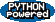PEAK-Rules/AST-Builder UserPreferences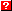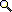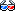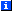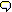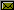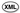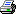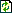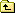# Building Expressions from Python Syntax Trees

The ast_builder module allows you to quickly navigate a Python syntax tree and perform operations on it. While Python 2.5 has a new "AST" feature that provides a high-level syntax tree, older Python versions offer a very low-level interface that provides complex tuple trees with lots of redundant information. The ast_builder module simplifies these trees dramatically, without creating an intermediate AST data structure (the way the stdlib compiler package does). Instead, it allows you to effectively "visit" a virtual AST structure and generate your desired output directly. In addition, it allows you to skip, delay, or repeat traversals of arbitrary subtrees.

This document describes the design (and tests the implementation) of the ast_builder module. You don't need to read it unless you want to use this module directly in your own programs. If you do want to use it directly, you should keep in mind that it currently only implements a subset of Python expression syntax: it does not support lambdas, yield expressions, or any kind of statements.

# Parse Trees and Builders

ast_builder operates on parse tuple trees, as created by the standard library parser module. The two API functions it provides are build and parse_expr:

```>>> from peak.rules.ast_builder import build, parse_expr
```

The build() function accepts two arguments, a "builder" and a "nodelist". A "builder" is an object that you supply that will perform actions on nodes in the parse tree. The "nodelist" is a parse tuple tree. As a shortcut, you can use parse_expr() to parse a string into a nodelist and invoke build() in one step.

A simple example:

```>>> class Builder:
...     def Const(self, const):
...         print const

>>> parse_expr("1", Builder())
1

>>> parse_expr("'foo'", Builder())
foo
```

As you can see, the builder's Const() method is invoked for integer and string constants, and it receives an actual value. Many other method names are used for more complex operations:

```>>> class Builder:
...     def Const(self, const):
...         return repr(const)
...         return "Add(%s, %s)" % (build(self,left), build(self,right))

>>> parse_expr("1+2", Builder())

>>> parse_expr("'foo'+'bar'", Builder())
```

Most builder methods accept nodelists as arguments. These nodelists can be recursively passed to build() in order to process expression subtrees. This is not done automatically, because it's possible you might want to skip processing of a particular subtree, or need to process a subtree with a different builder than the one currently in use, or even process a subtree with more than one builder (e.g. a builder that sees what names are bound within a function body, and a second builder to generate code).

For convenience in the rest of this document, we'll use a shorthand function to create a Builder(), parse an expression, and print the result:

```>>> def pe(expr):
...     print parse_expr(expr, Builder())
```

## Tokens

The Const and Name methods receive token values for constants and names respectively:

```>>> class Builder:
...     def Const(self, const):
...         return repr(const)
...
...     def Name(self, name):
...         return name

>>> pe("a")
a
>>> pe("b")
b
>>> pe("123")
123
>>> pe("'xyz'")
'xyz'
```

Note that adjacent string constants are automatically merged:

```>>> pe("'abc' 'xyz'")
'abcxyz'
```

## Unary Operators

There are five "unary" operator methods, that take a single argument: an AST tuple representing the expression the operator is applied to:

```>>> class Builder(Builder):
...     def unaryOp(fmt):
...         def method(self,expr):
...             return fmt % build(self,expr)
...         return method
...
...     UnaryPlus  = unaryOp('Plus(%s)')
...     UnaryMinus = unaryOp('Minus(%s)')
...     Invert     = unaryOp('Invert(%s)')
...     Backquote  = unaryOp('repr(%s)')
...     Not        = unaryOp('Not(%s)')

>>> pe("not - + ~`x`")
Not(Minus(Plus(Invert(repr(x)))))
```

## Attribute Access

The Getattr() method is called with a node and a string; the node is the base expression, and the string is the attribute that was accessed:

```>>> class Builder(Builder):
...     def Getattr(self, expr, attr):
...         return 'Getattr(%s,%r)' % (build(self,expr), attr)

>>> pe("a.b")
Getattr(a,'b')

>>> pe("a.b.c")
Getattr(Getattr(a,'b'),'c')
```

## Simple Binary Operators

There are 10 "simple" binary operator methods, that take a pair of left and right nodelists as arguments:

```>>> class Builder(Builder):
...     def mkBinOp(op):
...         pat = op + '(%s,%s)'
...         def method(self, left, right):
...             return pat % (build(self,left), build(self,right))
...         return method
...
...     Sub        = mkBinOp('Sub')
...     Mul        = mkBinOp('Mul')
...     Div        = mkBinOp('Div')
...     Mod        = mkBinOp('Mod')
...     FloorDiv   = mkBinOp('FloorDiv')
...     Power      = mkBinOp('Power')
...     LeftShift  = mkBinOp('LeftShift')
...     RightShift = mkBinOp('RightShift')
...     Subscript  = mkBinOp('Subscript')
```

Most of these operators correspond to normal Python binary operators:

```>>> pe("a+b")
>>> pe("b-a")
Sub(b,a)
>>> pe("c*d")
Mul(c,d)
>>> pe("c/d")
Div(c,d)
>>> pe("c%d")
Mod(c,d)
>>> pe("c//d")
FloorDiv(c,d)
>>> pe("a**b")
Power(a,b)
>>> pe("a<<b")
LeftShift(a,b)
>>> pe("a>>b")
RightShift(a,b)

>>> pe("a")
Subscript(a,1)
>>> pe("a")
Subscript(Subscript(a,1),2)
```

By the way, Ellipsis is also handled by the Const method, in the case where you have an expression like foo[...]:

```>>> pe("a[...]")
Subscript(a,Ellipsis)
```

## "List" Operators

The 7 "list operator" methods take a single argument: a sequence of nodes that represent a list of expressions delimited by the corresponding operator:

```>>> class Builder(Builder):
...     def multiOp(fmt,sep=','):
...         def method(self,items):
...             return fmt % sep.join([build(self,item) for item in items])
...         return method
...
...     And        = multiOp('And(%s)')
...     Or         = multiOp('Or(%s)')
...     Tuple      = multiOp('Tuple(%s)')
...     List       = multiOp('List(%s)')
...     Bitor      = multiOp('Bitor(%s)')
...     Bitxor     = multiOp('Bitxor(%s)')
...     Bitand     = multiOp('Bitand(%s)')

>>> pe("a and b")
And(a,b)
>>> pe("a or b")
Or(a,b)
>>> pe("a and b and c")
And(a,b,c)
>>> pe("a or b or c")
Or(a,b,c)
>>> pe("a and b and c and d")
And(a,b,c,d)
>>> pe("a or b or c or d")
Or(a,b,c,d)

>>> pe("a&b&c")
Bitand(a,b,c)
>>> pe("a|b|c")
Bitor(a,b,c)
>>> pe("a^b^c")
Bitxor(a,b,c)

>>> pe("a&b&c&d")
Bitand(a,b,c,d)
>>> pe("a|b|c|d")
Bitor(a,b,c,d)
>>> pe("a^b^c^d")
Bitxor(a,b,c,d)
```

### Tuples

No parens:

```>>> pe("a,")
Tuple(a)
>>> pe("a,b")
Tuple(a,b)
>>> pe("a,b,c")
Tuple(a,b,c)
>>> pe("a,b,c,")
Tuple(a,b,c)
```

With parens:

```>>> pe("()")
Tuple()
>>> pe("(a)")
a
>>> pe("(a,)")
Tuple(a)
>>> pe("(a,b)")
Tuple(a,b)
>>> pe("(a,b,)")
Tuple(a,b)
>>> pe("(a,b,c)")
Tuple(a,b,c)
>>> pe("(a,b,c,)")
Tuple(a,b,c)
```

### Lists

```>>> pe("[]")
List()
>>> pe("[a]")
List(a)
>>> pe("[a,]")
List(a)
>>> pe("[a,b]")
List(a,b)
>>> pe("[a,b,]")
List(a,b)
>>> pe("[a,b,c]")
List(a,b,c)
>>> pe("[a,b,c,]")
List(a,b,c)
```

## Slicing

The Slice2 method takes two arguments: a start and stop value. Each is either a node list or None (in which case there is no expression for that part of the slice):

```>>> class Builder(Builder):
...     def Slice2(self,start,stop):
...         txt = 'Slice('
...         if start:
...             txt += build(self, start)
...         txt += ':'
...         if stop:
...             txt += build(self, stop)
...         return txt+')'

>>> pe("a[:]")
Subscript(a,Slice(:))

>>> pe("a[1:2]")
Subscript(a,Slice(1:2))

>>> pe("a[1:]")
Subscript(a,Slice(1:))

>>> pe("a[:2]")
Subscript(a,Slice(:2))
```

The Slice3 method is similar, but takes three arguments:

```>>> class Builder(Builder):
...     def Slice3(self,start,stop,stride):
...         txt = 'Slice('
...         if start:
...             txt += build(self, start)
...         txt += ':'
...         if stop:
...             txt += build(self, stop)
...         txt += ':'
...         if stride:
...             txt += build(self, stride)
...         return txt+')'

>>> pe("a[::]")
Subscript(a,Slice(::))

>>> pe("a[1::]")
Subscript(a,Slice(1::))

>>> pe("a[:2:]")
Subscript(a,Slice(:2:))

>>> pe("a[1:2:]")
Subscript(a,Slice(1:2:))

>>> pe("a[::3]")
Subscript(a,Slice(::3))

>>> pe("a[1::3]")
Subscript(a,Slice(1::3))

>>> pe("a[:2:3]")
Subscript(a,Slice(:2:3))

>>> pe("a[1:2:3]")
Subscript(a,Slice(1:2:3))
```

## Comparisons and Conditional Expressions

The Compare method receives two arguments: a node for the first expression to be compared, followed by a list of (op, expr) tuples for subsequent comparisons. The op value is a string representing the comparison operator used, and each expr is a node:

```>>> class Builder(Builder):
...     def Compare(self,initExpr,comparisons):
...         data = [build(self,initExpr)]
...         for op,val in comparisons:
...             data.append(op)
...             data.append(build(self,val))
...         return 'Compare(%s)' % ' '.join(data)

>>> pe("a>b")
Compare(a > b)
>>> pe("a>=b")
Compare(a >= b)
>>> pe("a<b")
Compare(a < b)
>>> pe("a<=b")
Compare(a <= b)
>>> pe("a<>b")
Compare(a <> b)
>>> pe("a!=b")
Compare(a != b)
>>> pe("a==b")
Compare(a == b)
>>> pe("a in b")
Compare(a in b)
>>> pe("a is b")
Compare(a is b)
>>> pe("a not in b")
Compare(a not in b)
>>> pe("a is not b")
Compare(a is not b)
```

### N-Way Comparisons

If you don't want to have to process N-way comparisons in your builder, you can set the simplify_comparisons flag on your class to True, and N-way comparisons will be converted to and expressions:

```>>> Builder.simplify_comparisons = True

>>> pe("1<2<3")
And(Compare(1 < 2),Compare(2 < 3))
```

Otherwise, you can set the flag to False, and you will receive N-way comparisons as additional values in the list of (op, expr) tuples:

```>>> Builder.simplify_comparisons = False

>>> pe("1<2<3")
Compare(1 < 2 < 3)

>>> pe("a>=b>c<d")
Compare(a >= b > c < d)
```

Note that you must explicitly set simplify_comparisons to either a true or false value; there is no default.

### Conditional Expressions

The IfElse method receives three arguments: a node for the "true" value, a node for the condition, and a node for the "false" value:

```>>> class Builder(Builder):
...     def IfElse(self, trueVal, condition, falseVal):
...         return 'IfElse(%s, %s, %s)' % (
...             build(self, trueVal), build(self, condition),
...             build(self, falseVal)
...         )

>>> import sys
>>> if sys.version>='2.5':
...     pe("a if b else c")
... else:
...     print "IfElse(a, b, c)"
IfElse(a, b, c)
```

## List Comprehensions and Generator Expressions

The ListComp and GenExpr methods receive two arguments: a node for the output expression, and a list of (op, node) tuples, where op is the name of an operator (either "for", "in", or "if"), and node is the node corresponding to the operator's argument:

```>>> class Builder(Builder):
...     def ListComp(self, initExpr, clauses):
...         data = [build(self,initExpr)]
...         for op,val in clauses:
...             data.append(op)
...             data.append(build(self,val))
...         return 'ListComp(%s)' % ' '.join(data)
...
...     def GenExpr(self, initExpr, clauses):
...         data = [build(self,initExpr)]
...         for op,val in clauses:
...             data.append(op)
...             data.append(build(self,val))
...         return 'GenExpr(%s)' % ' '.join(data)

>>> pe("[x for x in y if z]")
ListComp(x for x in y if z)

>>> pe("[(x+1, 42) for x in y for y in z if q>z]")
ListComp(Tuple(Add(x,1),42) for x in y for y in z if Compare(q > z))

>>> pe("[x+1 for x in y if x in z for y in q if r if p]")
ListComp(Add(x,1) for x in y if Compare(x in z) for y in q if r if p)

>>> if sys.version>='2.4':
...     pe("(x for x in y if z)")
... else:
...     print "GenExpr(x for x in y if z)"
GenExpr(x for x in y if z)
```

Note, by the way, that when you are building the "for" clause assignments, you'll need to handle arbitrary assignments (e.g. tuple unpacking):

```>>> pe("[x for y, x in z]")
ListComp(x for Tuple(y,x) in z)

>>> pe("[x.y for x.y in z]")
ListComp(Getattr(x,'y') for Getattr(x,'y') in z)

>>> pe("[x[y] for x[y] in z]")
ListComp(Subscript(x,y) for Subscript(x,y) in z)
```

(Normally, you would handle this by passing the "for" clauses to a different builder instance that's set up to handle calls to Name, Getattr, Tuple, etc. by generating assignments instead of lookups.)

## Dictionaries

The Dict method takes one argument: a list of (key, value) tuples, where both the keys and values are expression nodes:

```>>> class Builder(Builder):
...     def Dict(self, items):
...         return '{%s}' % ','.join([
...             '%s:%s' % (build(self,k),build(self,v)) for k,v in items
...         ])

>>> pe("{ (a,b):c+d, e:[f,g]  }")
```

## Calls

The CallFunc method takes five arguments:

func
The expression to be called.
args
A list of positional argument expression nodes.
kw
A list of (argname, value) expression node pairs
star_node
The node for the *args expression, or None if there isn't one.
dstar_node

The node for the *kw expression, or None if there isn't one.

```>>> class Builder(Builder):
...     def CallFunc(self, func, args, kw, star_node, dstar_node):
...         if star_node:
...             star_node=build(self, star_node)
...         else:
...             star_node = 'None'
...         if dstar_node:
...             dstar_node=build(self, dstar_node)
...         else:
...             dstar_node = 'None'
...         return 'Call(%s,%s,%s,%s,%s)' % (
...             build(self,func), self.Tuple(args), self.Dict(kw),
...             star_node, dstar_node
...         )
```
```>>> pe("a()")
Call(a,Tuple(),{},None,None)
```
```>>> pe("a(1,2)")
Call(a,Tuple(1,2),{},None,None)
>>> pe("a(1,2,)")
Call(a,Tuple(1,2),{},None,None)
>>> pe("a(b=3)")
Call(a,Tuple(),{'b':3},None,None)
>>> pe("a(1,2,b=3)")
Call(a,Tuple(1,2),{'b':3},None,None)
```
```>>> pe("a(*x)")
Call(a,Tuple(),{},x,None)
>>> pe("a(1,*x)")
Call(a,Tuple(1),{},x,None)
>>> pe("a(b=3,*x)")
Call(a,Tuple(),{'b':3},x,None)
>>> pe("a(1,2,b=3,*x)")
Call(a,Tuple(1,2),{'b':3},x,None)
```
```>>> pe("a(**y)")
Call(a,Tuple(),{},None,y)
>>> pe("a(1,**y)")
Call(a,Tuple(1),{},None,y)
>>> pe("a(b=3,**y)")
Call(a,Tuple(),{'b':3},None,y)
>>> pe("a(1,2,b=3,**y)")
Call(a,Tuple(1,2),{'b':3},None,y)
```
```>>> pe("a(*x,**y)")
Call(a,Tuple(),{},x,y)
>>> pe("a(1,*x,**y)")
Call(a,Tuple(1),{},x,y)
>>> pe("a(b=3,*x,**y)")
Call(a,Tuple(),{'b':3},x,y)
>>> pe("a(1,2,b=3,*x,**y)")
Call(a,Tuple(1,2),{'b':3},x,y)
```
```>>> if sys.version>='2.4':
...     pe("a(x for x in y if z)")
...     pe("a(x for x in y if z, q)")
... else:
...     print "Call(a,Tuple(GenExpr(x for x in y if z)),{},None,None)"
...     print "Call(a,Tuple(GenExpr(x for x in y if z),q),{},None,None)"
Call(a,Tuple(GenExpr(x for x in y if z)),{},None,None)
Call(a,Tuple(GenExpr(x for x in y if z),q),{},None,None)
```

## Miscellaneous Tests

An interesting quirk of the AST module is that it supports parsing some calls that should be syntax errors. The ast_builder module thus has to trap these itself:

```>>> pe("a(1=2)")    # expr as kw
Traceback (most recent call last):
...
SyntaxError: keyword can't be an expression (...)

>>> pe("a(b=2,c)")
Traceback (most recent call last):
...
SyntaxError: non-keyword arg after keyword arg
```

Most of Python's operator associativity and precedence is grammar-driven, but certain parts have to be handled by ast_builder. These are just some tests to make sure that associativity is correct:

```>>> pe("a+b+c")
>>> pe("a*b*c")
Mul(Mul(a,b),c)
>>> pe("a/b/c")
Div(Div(a,b),c)
>>> pe("a//b//c")
FloorDiv(FloorDiv(a,b),c)
>>> pe("a%b%c")
Mod(Mod(a,b),c)
>>> pe("a<<b<<c")
LeftShift(LeftShift(a,b),c)
>>> pe("a>>b>>c")
RightShift(RightShift(a,b),c)
>>> pe("a()()")
Call(Call(a,Tuple(),{},None,None),Tuple(),{},None,None)

>>> pe("a**b**c")   # power is right-associative
Power(a,Power(b,c))

>>> pe("5*x**2 + 4*x + -1")